登  录风  格绿草青青青青苹果蓝色经典佳人有约蓝色梦想蓝天白云蓝色狂想蓝色魅力魅力星空
 »  游客当前位置：  论坛首页 »  自由讨论区 »  AI.人工智能讨论区 »总帖数 1 每页帖数 10 1/1页 1
0查看: 1136 | 回复: 0 主题： [转帖]AI从入门到放弃：BP神经网络算法推导及代码实现笔记huang.wang 高级会员等级：中将 经验：17601 发帖：406 精华：1 注册：1970-1-1 状态：离线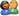加好友发消息

 本文转自 知乎专栏 腾讯码农一. 前言作为AI入门小白，参考了一些文章，想记点笔记加深印象，发出来是给有需求的童鞋学习共勉，大神轻拍！【毒鸡汤】：算法这东西，读完之后的状态多半是 --> “我是谁，我在哪？” 没事的，吭哧吭哧学总能学会，毕竟还有千千万万个算法等着你。本文货很干，堪比沙哈拉大沙漠，自己挑的文章，含着泪也要读完！ 二. 科普1、生物上的神经元就是接收四面八方的刺激（输入），然后做出反应（输出），给它一点阳光就灿烂。2、仿生嘛，于是喜欢放飞自我的 某些人 就提出了人工神经网络。一切的基础-->人工神经单元，看图：三. 通往沙漠的入口: 神经元是什么，有什么用开始前，需要搞清楚一个很重要的问题：人工神经网络里的神经元是什么，有什么用。只有弄清楚这个问题，你才知道你在哪里，在做什么，要往哪里去。首先，回顾一下神经元的结构，看下图, 我们先忽略激活函数不管：没错，开始晒公式了！我们的数据都是离散的，为了看得更清楚点，所以换个表达方式，把离散的数据写成向量。该不会忘了向量是啥吧？回头致电问候一下当年的体育老师！现在回答问题刚才的问题：1、一个神经元是什么：参照式（1.6），从函数图像角度看，这就是一根直线。2、一个神经元有什么用：要说明用途就要给出一个应用场景：分类。一个神经元就是一条直线，相当于楚河汉界，可以把红棋绿棋分隔开，此时它就是个分类器。所以，在线性场景下，单个神经元能达到分类的作用，它总能学习到一条合适的直线，将两类元素区分出来。先睹为快，看效果图，自己可以去玩，戳我直达。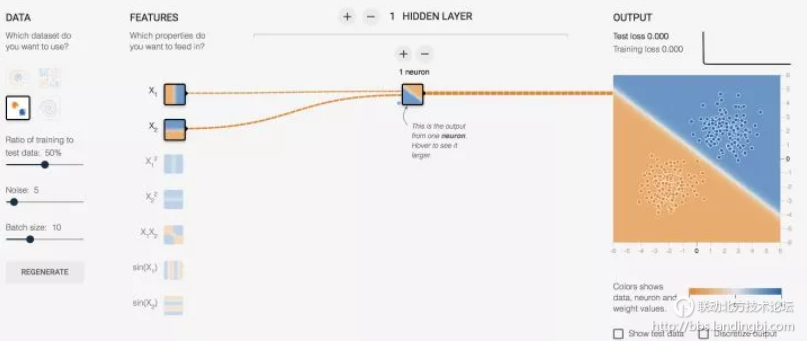对上面的图简单说明一下——(x1,x2) 对于神经元的输入都是 x, 而对我们而言，这数据就是意义上的点的坐标，我们习惯写成 (x,y)。又要划重点了：我们需要对神经元的输出做判定，那么就需要有判定规则，通过判定规则后我们才能拿到我们想要的结果，这个规则就是：1、假设0代表红点，1代表蓝点（这些数据都是事先标定好的，在监督学习下，神经元会知道点是什么颜色并以这个已知结果作为标杆进行学习）；2、当神经元输出小于等于 0 时，最终结果输出为 0，这是个红点；3、当神经元输出大于 1 时，最终结果输出为 1，这是个蓝点上面提到的规则让我闻到了激活函数的味道！（这里只是线性场景，虽然不合适，但是简单起见，使用了单位阶跃函数来描述激活函数的功能）当 x<=0 时，y = 0; 当 x > 0 时，y = 1这是阶跃函数的长相：此时神经元的长相：四. 茫茫大漠第一步: 激活函数是什么，有什么用从上面的例子，其实已经说明了激活函数的作用；但是，我们通常面临的问题，不是简单的线性问题，不能用单位阶跃函数作为激活函数，原因是：阶跃函数在x=0时不连续，即不可导，在非0处导数为0。用人话说就是它具备输出限定在[0-1]，但是它不具备丝滑的特性，这个特性很重要。并且在非0处导数为0，也就是硬饱和，压根儿就没梯度可言，梯度也很重要，梯度意味着在神经元传播间是有反应的，而不是“死”了的。接下来说明下，激活函数所具备的特性有什么，只挑重要的几点特性讲：    非线性: 即导数不是常数,不然就退化成直线。对于一些画一条直线仍然无法分开的问题，非线性可以把直线掰弯，自从变弯以后，就能包罗万象了。    几乎处处可导：也就是具备“丝滑的特性”，不要应激过度，要做正常人。数学上，处处可导为后面降到的后向传播算法（BP算法）提供了核心条件    输出范围有限：一般是限定在[0,1]，有限的输出范围使得神经元对于一些比较大的输入也会比较稳定。    非饱和性：饱和就是指，当输入比较大的时候，输出几乎没变化了，那么会导致梯度消失！什么是梯度消失：就是你天天给女生送花，一开始妹纸还惊喜，到后来直接麻木没反应了。梯度消失带来的负面影响就是会限制了神经网络表达能力，词穷的感觉你有过么。sigmod，tanh函数都是软饱和的，阶跃函数是硬饱和。软是指输入趋于无穷大的时候输出无限接近上线，硬是指像阶跃函数那样，输入非0输出就已经始终都是上限值。数学表示我就懒得写了，传送门在此（https://www.cnblogs.com/rgvb178/p/6055213.html）,里面有写到。如果激活函数是饱和的，带来的缺陷就是系统迭代更新变慢，系统收敛就慢，当然这是可以有办法弥补的，一种方法是使用交叉熵函数作为损失函数，这里不多说。ReLU是非饱和的，亲测效果挺不错，所以这货最近挺火的。    单调性：即导数符号不变。导出要么一直大于0，要么一直小于0，不要上蹿下跳。导数符号不变，让神经网络训练容易收敛。这里只说我们用到的激活函数：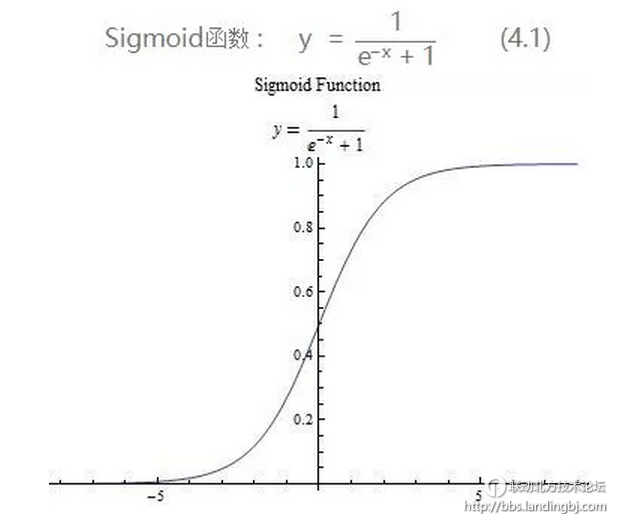求一下它的导数把，因为后面讲bp算法会直接套用它：先祭出大杀器，高中数学之复合函数求导法则：它的导数图像：五. 沙漠中心的风暴：BP(Back Propagation)算法1. 神经网络的结构经过上面的介绍，单个神经元不足以让人心动，唯有组成网络。神经网络是一种分层结构，一般由输入曾，隐藏层，输出层组成。所以神经网络至少有3层，隐藏层多于1，总层数大于3的就是我们所说的深度学习了。    输入层：就是接收原始数据，然后往隐层送    输出层：神经网络的决策输出    隐藏层：该层可以说是神经网络的关键，相当于对数据做一次特征提取。隐藏层的意义，是把前一层的向量变成新的向量。就是坐标变换，说人话就是把数据做平移，旋转，伸缩，扭曲，让数据变得线性可分。可能这个不那么好理解，举个栗子：下面的图左侧是原始数据，中间很多绿点，外围是很多红点，如果你是神经网络，你会怎么做呢？一种做法：把左图的平面看成一块布，把它缝合成一个闭合的包包（相当于数据变换到了一个3维坐标空间），然后把有绿色点的部分撸到顶部（伸缩和扭曲），然后外围的红色点自然在另一端了，要是姿势还不够帅，就挪挪位置（平移）。这时候干脆利落的砍一刀，绿点红点就彻底区分开了。重要的东西再说一遍：神经网络换着坐标空间玩数据，根据需要，可降维，可升维，可大，可小，可圆可扁，就是这么“无敌”2.正反向传播过程看图，这是一个典型的三层神经网络结构，第一层是输入层，第二层是隐藏层，第三层是输出层。PS:不同的应用场景，神经网络的结构要有针对性的设计，这里仅仅是为了推导算法和计算方便才采用这个简单的结构。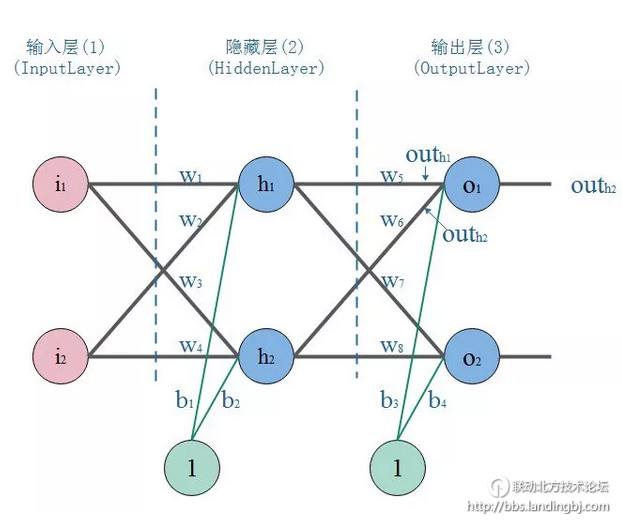我们以战士打靶，目标是训练战士能命中靶心成为神枪手作为场景：那么我们手里有这样一些数据：一堆枪摆放的位置(x,y)，以及射击结果，命中靶心和不命中靶心。我们的目标是：训练出一个神经网络模型，输入一个点的坐标（射击姿势），它就告诉你这个点是什么结果（是否命中）。我们的方法是：训练一个能根据误差不断自我调整的模型，训练模型的步骤是：（1）正向传播：把点的坐标数据输入神经网络，然后开始一层一层的传播下去，直到输出层输出结果。（2）反向传播(BP)：就好比战士去靶场打靶，枪的摆放位置（输入），和靶心（期望的输出）是已知。战士（神经网络）一开始的时候是这样做的，随便开一枪（w，b参数初始化称随机值），观察结果（这时候相当于进行了一次正向传播）。然后发现，偏离靶心左边，应该往右点儿打。所以战士开始根据偏离靶心的距离（误差，也称损失）调整了射击方向往右一点（这时，完成了一次反向传播）（3）当完成了一次正反向传播，也就完成了一次神经网络的训练迭代，反复调整射击角度（反复迭代），误差越来越小，战士打得越来越准，神枪手模型也就诞生了。3.BP算法推导和计算参数初始化：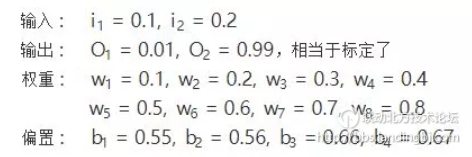正向传播：2.隐层-->输出层：正向传播结束，我们看看输出层的输出结果：[0.7987314002, 0.8374488853]，但是我们希望它能输出[0.01, 0.99]，所以明显的差太远了，这个时候我们就需要利用反向传播，更新权值w，然后重新计算输出。反向传播1.计算输出误差：PS: 这里我要说的是，用这个作为误差的计算，因为它简单，实际上用的时候效果不咋滴。如果激活函数是饱和的，带来的缺陷就是系统迭代更新变慢，系统收敛就慢，当然这是可以有办法弥补的，一种方法是使用交叉熵函数作为损失函数。交叉熵做为代价函数能达到上面说的优化系统收敛下欧工，是因为它在计算误差对输入的梯度时，抵消掉了激活函数的导数项，从而避免了因为激活函数的“饱和性”给系统带来的负面影响。如果项了解更详细的证明可以戳我直达。对输出的偏导数：2.隐层-->输出层的权值及偏置b的更新：先放出链式求导法则：以更新w5举例：我们知道，权重w的大小能直接影响输出，w不合适那么会使得输出误差。要想直到某一个w值对误差影响的程度，可以用误差对该w的变化率来表达。如果w的一点点变动，就会导致误差增大很多，说明这个w对误差影响的程度就更大，也就是说，误差对该w的变化率越高。而误差对w的变化率就是误差对w的偏导。所以，看下图，总误差的大小首先受输出层神经元O1的输出影响，继续反推，O1的输出受它自己的输入的影响，而它自己的输入会受到w5的影响。这就是连锁反应，从结果找根因。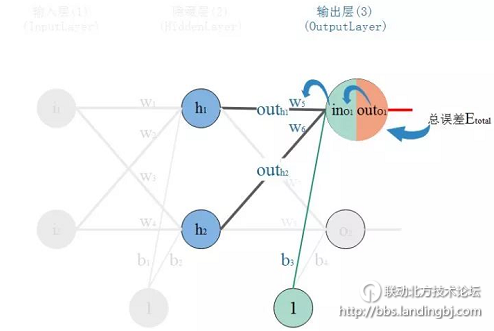那么，根据链式法则则有：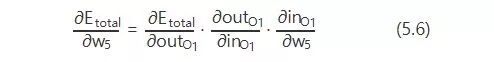现在挨个计算：有个学习率的东西，学习率取个0.5。关于学习率，不能过高也不能过低。因为训练神经网络系统的过程，就是通过不断的迭代，找到让系统输出误差最小的参数的过程。每一次迭代都经过反向传播进行梯度下降，然而误差空间不是一个滑梯，一降到底，常规情况下就像坑洼的山地。学习率太小，那就很容易陷入局部最优，就是你认为的最低点并不是整个空间的最低点。如果学习率太高，那系统可能难以收敛，会在一个地方上串下跳，无法对准目标（目标是指误差空间的最低点），可以看图：xy轴是权值w平面，z轴是输出总误差。整个误差曲面可以看到两个明显的低点，显然右边最低，属于全局最优。而左边的是次低，从局部范围看，属于局部最优。而图中，在给定初始点的情况下，标出的两条抵达低点的路线，已经是很理想情况的梯度下降路径。3.输入层-->隐层的权值及偏置b更新：以更新w1为例：仔细观察，我们在求w5的更新，误差反向传递路径输出层-->隐层，即out(O1)-->in(O1)-->w5，总误差只有一根线能传回来。但是求w1时，误差反向传递路径是隐藏层-->输入层，但是隐藏层的神经元是有2根线的，所以总误差沿着2个路径回来，也就是说，计算偏导时，要分开来算。看图：那么，现在开始算总误差对w1的偏导：4.结论：我们通过亲力亲为的计算，走过了正向传播，也体会了反向传播，完成了一次训练(迭代)。随着迭代加深，输出层的误差会越来越小，专业点说就是系统趋于收敛。来一张系统误差随迭代次数变化的图来表明我刚才说描述：六. 沙漠的绿洲：代码实现1. 代码代码！其实已经有很多机器学习的框架可以很简单的实现神经网络。但是我们的目标是：在看懂算法之后，我们是否能照着算法的整个过程，去实现一遍，可以加深对算法原理的理解，以及对算法实现思路的的理解。顺便说打个call，numpy这个库，你值得拥有！代码实现如下。代码里已经做了尽量啰嗦的注释，关键实现的地方对标了公式的编号，如果看的不明白的地方多回来啃一下算法推导。对应代码也传到了github上。代码能自己定义神经网络的结构，支持深度网络。代码实现了对红蓝颜色的点做分类的模型训练，通过3层网络结构，改变隐藏层的神经元个数，通过图形显示隐藏层神经元数量对问题的解释能力。代码中还实现了不同激活函数。隐藏层可以根据需要换着激活函数玩，输出层一般就用sigmoid，当然想换也随你喜欢～```#coding:utf-8 import h5py import sklearn.datasets import sklearn.linear_model import matplotlib import matplotlib.font_manager as fm import matplotlib.pyplot as plt import numpy as np np.random.seed(1) font = fm.FontProperties(fname='/System/Library/Fonts/STHeiti Light.ttc') matplotlib.rcParams['figure.figsize'] = (10.0, 8.0) def sigmoid(input_sum):     """     函数：         激活函数Sigmoid     输入：         input_sum: 输入，即神经元的加权和     返回：         output: 激活后的输出         input_sum: 把输入缓存起来返回     """     output = 1.0/(1+np.exp(-input_sum))     return output, input_sum def sigmoid_back_propagation(derror_wrt_output, input_sum):     """     函数：         误差关于神经元输入的偏导: dE／dIn = dE/dOut * dOut/dIn  参照式（5.6）         其中： dOut/dIn 就是激活函数的导数 dy=y(1 - y)，见式（5.9）               dE/dOut 误差对神经元输出的偏导，见式（5.8）     输入：         derror_wrt_output：误差关于神经元输出的偏导: dE/dyⱼ = 1/2(d(expect_to_output - output)**2/doutput) = -(expect_to_output - output)         input_sum: 输入加权和     返回：         derror_wrt_dinputs: 误差关于输入的偏导，见式（5.13）     """     output = 1.0/(1 + np.exp(- input_sum))     doutput_wrt_dinput = output * (1 - output)     derror_wrt_dinput =  derror_wrt_output * doutput_wrt_dinput     return derror_wrt_dinput def relu(input_sum):     """         函数：             激活函数ReLU         输入：             input_sum: 输入，即神经元的加权和         返回：             outputs: 激活后的输出             input_sum: 把输入缓存起来返回     """     output = np.maximum(0, input_sum)     return output, input_sum def relu_back_propagation(derror_wrt_output, input_sum):     """         函数：             误差关于神经元输入的偏导: dE／dIn = dE/dOut * dOut/dIn             其中： dOut/dIn 就是激活函数的导数                   dE/dOut 误差对神经元输出的偏导         输入：             derror_wrt_output：误差关于神经元输出的偏导             input_sum: 输入加权和         返回：             derror_wrt_dinputs: 误差关于输入的偏导     """     derror_wrt_dinputs = np.array(derror_wrt_output, copy=True)     derror_wrt_dinputs[input_sum <= 0] = 0     return derror_wrt_dinputs def tanh(input_sum):     """     函数：         激活函数 tanh     输入：         input_sum: 输入，即神经元的加权和     返回：         output: 激活后的输出         input_sum: 把输入缓存起来返回     """     output = np.tanh(input_sum)     return output, input_sum def tanh_back_propagation(derror_wrt_output, input_sum):     """     函数：         误差关于神经元输入的偏导: dE／dIn = dE/dOut * dOut/dIn         其中： dOut/dIn 就是激活函数的导数 tanh'(x) = 1 - x²               dE/dOut 误差对神经元输出的偏导     输入：         derror_wrt_output：误差关于神经元输出的偏导: dE/dyⱼ = 1/2(d(expect_to_output - output)**2/doutput) = -(expect_to_output - output)         input_sum: 输入加权和     返回：         derror_wrt_dinputs: 误差关于输入的偏导     """     output = np.tanh(input_sum)     doutput_wrt_dinput = 1 - np.power(output, 2)     derror_wrt_dinput =  derror_wrt_output * doutput_wrt_dinput     return derror_wrt_dinput def activated(activation_choose, input):     """把正向激活包装一下"""     if activation_choose == "sigmoid":         return sigmoid(input)     elif activation_choose == "relu":         return relu(input)     elif activation_choose == "tanh":         return tanh(input)     return sigmoid(input) def activated_back_propagation(activation_choose, derror_wrt_output, output):     """包装反向激活传播"""     if activation_choose == "sigmoid":         return sigmoid_back_propagation(derror_wrt_output, output)     elif activation_choose == "relu":         return relu_back_propagation(derror_wrt_output, output)     elif activation_choose == "tanh":         return tanh_back_propagation(derror_wrt_output, output)     return sigmoid_back_propagation(derror_wrt_output, output) class NeuralNetwork:     def __init__(self, layers_strcuture, print_cost = False):         self.layers_strcuture = layers_strcuture         self.layers_num = len(layers_strcuture)         # 除掉输入层的网络层数，因为其他层才是真正的神经元层         self.param_layers_num = self.layers_num - 1         self.learning_rate = 0.0618         self.num_iterations = 2000         self.x = None         self.y = None         self.w = dict()         self.b = dict()         self.costs = []         self.print_cost = print_cost         self.init_w_and_b()     def set_learning_rate(self, learning_rate):         """设置学习率"""         self.learning_rate = learning_rate     def set_num_iterations(self, num_iterations):         """设置迭代次数"""         self.num_iterations = num_iterations     def set_xy(self, input, expected_output):         """设置神经网络的输入和期望的输出"""         self.x = input         self.y = expected_output     def init_w_and_b(self):         """         函数:             初始化神经网络所有参数         输入:             layers_strcuture: 神经网络的结构，例如[2,4,3,1]，4层结构:                 第0层输入层接收2个数据，第1层隐藏层4个神经元，第2层隐藏层3个神经元，第3层输出层1个神经元         返回: 神经网络各层参数的索引表，用来定位权值 wᵢ  和偏置 bᵢ，i为网络层编号         """         np.random.seed(3)         # 当前神经元层的权值为 n_i x n_(i-1)的矩阵，i为网络层编号，n为下标i代表的网络层的节点个数         # 例如[2,4,3,1]，4层结构：第0层输入层为2，那么第1层隐藏层神经元个数为4         # 那么第1层的权值w是一个 4x2 的矩阵，如：         #    w1 = array([ [-0.96927756, -0.59273074],         #                 [ 0.58227367,  0.45993021],         #                 [-0.02270222,  0.13577601],         #                 [-0.07912066, -1.49802751] ])         # 当前层的偏置一般给0就行，偏置是个1xnᵢ的矩阵，nᵢ为第i层的节点个数，例如第1层为4个节点，那么：         #    b1 = array([ 0.,  0.,  0.,  0.])         for l in range(1, self.layers_num):             self.w["w" + str(l)] = np.random.randn(self.layers_strcuture[l], self.layers_strcuture[l-1])/np.sqrt(self.layers_strcuture[l-1])             self.b["b" + str(l)] = np.zeros((self.layers_strcuture[l], 1))         return self.w, self.b     def layer_activation_forward(self, x, w, b, activation_choose):         """         函数：             网络层的正向传播         输入：             x: 当前网络层输入（即上一层的输出），一般是所有训练数据，即输入矩阵             w: 当前网络层的权值矩阵             b: 当前网络层的偏置矩阵             activation_choose: 选择激活函数 "sigmoid", "relu", "tanh"         返回:             output: 网络层的激活输出             cache: 缓存该网络层的信息，供后续使用： (x, w, b, input_sum) -> cache      """         # 对输入求加权和，见式（5.1）         input_sum = np.dot(w, x) + b         # 对输入加权和进行激活输出         output, _ = activated(activation_choose, input_sum)         return output, (x, w, b, input_sum)     def forward_propagation(self, x):         """         函数:             神经网络的正向传播         输入:         返回:             output: 正向传播完成后的输出层的输出             caches: 正向传播过程中缓存每一个网络层的信息： (x, w, b, input_sum),... -> caches         """         caches = []         #作为输入层，输出 = 输入         output_prev = x         #第0层为输入层，只负责观察到输入的数据，并不需要处理，正向传播从第1层开始，一直到输出层输出为止         # range(1, n) => [1, 2, ..., n-1]         L = self.param_layers_num         for l in range(1, L):             # 当前网络层的输入来自前一层的输出             input_cur = output_prev             output_prev, cache = self.layer_activation_forward(input_cur, self.w["w"+ str(l)], self.b["b" + str(l)], "tanh")             caches.append(cache)         output, cache = self.layer_activation_forward(output_prev, self.w["w" + str(L)], self.b["b" + str(L)], "sigmoid")         caches.append(cache)         return output, caches     def show_caches(self, caches):         """显示网络层的缓存参数信息"""         i = 1         for cache in caches:             print("%dtd Layer" % i)             print(" input: %s" % cache)             print(" w: %s" % cache)             print(" b: %s" % cache)             print(" input_sum: %s" % cache)             print("----------")             i += 1     def compute_error(self, output):         """         函数:             计算档次迭代的输出总误差         输入:         返回:         """         m = self.y.shape         # 计算误差，见式(5.5): E = Σ1/2(期望输出-实际输出)²         #error = np.sum(0.5 * (self.y - output) ** 2) / m         # 交叉熵作为误差函数         error =  -np.sum(np.multiply(np.log(output),self.y) + np.multiply(np.log(1 - output), 1 - self.y)) / m         error = np.squeeze(error)         return error     def layer_activation_backward(self, derror_wrt_output, cache, activation_choose):         """             函数:                 网络层的反向传播             输入:                 derror_wrt_output: 误差关于输出的偏导                 cache: 网络层的缓存信息 (x, w, b, input_sum)                 activation_choose: 选择激活函数 "sigmoid", "relu", "tanh"             返回: 梯度信息，即                 derror_wrt_output_prev: 反向传播到上一层的误差关于输出的梯度                 derror_wrt_dw: 误差关于权值的梯度                 derror_wrt_db: 误差关于偏置的梯度         """         input, w, b, input_sum = cache         output_prev = input     # 上一层的输出 = 当前层的输入; 注意是'输入'不是输入的加权和（input_sum）         m = output_prev.shape      # m是输入的样本数量，我们要取均值，所以下面的求值要除以m         # 实现式（5.13）-> 误差关于权值w的偏导数         derror_wrt_dinput = activated_back_propagation(activation_choose, derror_wrt_output, input_sum)         derror_wrt_dw = np.dot(derror_wrt_dinput, output_prev.T) / m         # 实现式 （5.32）-> 误差关于偏置b的偏导数         derror_wrt_db = np.sum(derror_wrt_dinput, axis=1, keepdims=True)/m         # 为反向传播到上一层提供误差传递，见式（5.28）的 （Σδ·w） 部分         derror_wrt_output_prev = np.dot(w.T, derror_wrt_dinput)         return derror_wrt_output_prev, derror_wrt_dw, derror_wrt_db     def back_propagation(self, output, caches):         """         函数:             神经网络的反向传播         输入:             output：神经网络输             caches：所有网络层（输入层不算）的缓存参数信息  [(x, w, b, input_sum), ...]         返回:             grads: 返回当前迭代的梯度信息         """         grads = {}         L = self.param_layers_num #         output = output.reshape(output.shape)  # 把输出层输出输出重构成和期望输出一样的结构         expected_output = self.y         # 见式(5.8)         #derror_wrt_output = -(expected_output - output)         # 交叉熵作为误差函数         derror_wrt_output = - (np.divide(expected_output, output) - np.divide(1 - expected_output, 1 - output))         # 反向传播：输出层 -> 隐藏层，得到梯度：见式(5.8), (5.13), (5.15)         current_cache = caches[L - 1] # 取最后一层,即输出层的参数信息         grads["derror_wrt_output" + str(L)], grads["derror_wrt_dw" + str(L)], grads["derror_wrt_db" + str(L)] = \             self.layer_activation_backward(derror_wrt_output, current_cache, "sigmoid")         # 反向传播：隐藏层 -> 隐藏层，得到梯度：见式 (5.28)的(Σδ·w), (5.28), (5.32)         for l in reversed(range(L - 1)):             current_cache = caches[l]             derror_wrt_output_prev_temp, derror_wrt_dw_temp, derror_wrt_db_temp = \                 self.layer_activation_backward(grads["derror_wrt_output" + str(l + 2)], current_cache, "tanh")             grads["derror_wrt_output" + str(l + 1)] = derror_wrt_output_prev_temp             grads["derror_wrt_dw" + str(l + 1)] = derror_wrt_dw_temp             grads["derror_wrt_db" + str(l + 1)] = derror_wrt_db_temp         return grads     def update_w_and_b(self, grads):         """         函数:             根据梯度信息更新w，b         输入:             grads：当前迭代的梯度信息         返回:         """         # 权值w和偏置b的更新，见式:（5.16),(5.18)         for l in range(self.param_layers_num):             self.w["w" + str(l + 1)] = self.w["w" + str(l + 1)] - self.learning_rate * grads["derror_wrt_dw" + str(l + 1)]             self.b["b" + str(l + 1)] = self.b["b" + str(l + 1)] - self.learning_rate * grads["derror_wrt_db" + str(l + 1)]     def training_modle(self):         """训练神经网络模型"""         np.random.seed(5)         for i in range(0, self.num_iterations):             # 正向传播，得到网络输出，以及每一层的参数信息             output, caches = self.forward_propagation(self.x)             # 计算网络输出误差             cost = self.compute_error(output)             # 反向传播，得到梯度信息             grads = self.back_propagation(output, caches)             # 根据梯度信息，更新权值w和偏置b             self.update_w_and_b(grads)             # 当次迭代结束，打印误差信息             if self.print_cost and i % 1000 == 0:                 print ("Cost after iteration %i: %f" % (i, cost))             if self.print_cost and i % 1000 == 0:                 self.costs.append(cost)         # 模型训练完后显示误差曲线         if False:             plt.plot(np.squeeze(self.costs))             plt.ylabel(u'神经网络误差', fontproperties = font)             plt.xlabel(u'迭代次数 (*100)', fontproperties = font)             plt.title(u"学习率 =" + str(self.learning_rate), fontproperties = font)             plt.show()         return self.w, self.b     def predict_by_modle(self, x):         """使用训练好的模型（即最后求得w，b参数）来决策输入的样本的结果"""         output, _ = self.forward_propagation(x.T)         output = output.T         result = output / np.sum(output, axis=1, keepdims=True)         return np.argmax(result, axis=1) def plot_decision_boundary(xy, colors, pred_func):     # xy是坐标点的集合，把集合的范围算出来     # 加减0.5相当于扩大画布的范围，不然画出来的图坐标点会落在图的边缘，逼死强迫症患者     x_min, x_max = xy[:, 0].min() - 0.5, xy[:, 0].max() + 0.5     y_min, y_max = xy[:, 1].min() - 0.5, xy[:, 1].max() + 0.5     # 以h为分辨率，生成采样点的网格，就像一张网覆盖所有颜色点     h = .01     xx, yy = np.meshgrid(np.arange(x_min, x_max, h), np.arange(y_min, y_max, h))     # 把网格点集合作为输入到模型，也就是预测这个采样点是什么颜色的点，从而得到一个决策面     Z = pred_func(np.c_[xx.ravel(), yy.ravel()])     Z = Z.reshape(xx.shape)     # 利用等高线，把预测的结果画出来，效果上就是画出红蓝点的分界线     plt.contourf(xx, yy, Z, cmap=plt.cm.Spectral)     # 训练用的红蓝点点也画出来     plt.scatter(xy[:, 0], xy[:, 1], c=colors, marker='o', cmap=plt.cm.Spectral, edgecolors='black') if __name__ == "__main__":     plt.figure(figsize=(16, 32))     # 用sklearn的数据样本集，产生2种颜色的坐标点，noise是噪声系数，噪声越大，2种颜色的点分布越凌乱     xy, colors = sklearn.datasets.make_moons(60, noise=1.0)     # 因为点的颜色是1bit，我们设计一个神经网络，输出层有2个神经元。     # 标定输出[1,0]为红色点，输出[0,1]为蓝色点     expect_output = []     for c in colors:         if c == 1:             expect_output.append([0,1])         else:             expect_output.append([1,0])     expect_output = np.array(expect_output).T     # 设计3层网络，改变隐藏层神经元的个数，观察神经网络分类红蓝点的效果     hidden_layer_neuron_num_list = [1,2,4,10,20,50]     for i, hidden_layer_neuron_num in enumerate(hidden_layer_neuron_num_list):         plt.subplot(5, 2, i + 1)         plt.title(u'隐藏层神经元数量: %d' % hidden_layer_neuron_num, fontproperties = font)         nn = NeuralNetwork([2, hidden_layer_neuron_num, 2], True)         # 输出和输入层都是2个节点，所以输入和输出的数据集合都要是 nx2的矩阵         nn.set_xy(xy.T, expect_output)         nn.set_num_iterations(30000)         nn.set_learning_rate(0.1)         w, b = nn.training_modle()         plot_decision_boundary(xy, colors, nn.predict_by_modle)     plt.show()```2. 晒图晒图！关于误差曲线(这里只举其中一个栗子)：通过看误差曲线，可以从一定程度上判定网络的效果，模型训练是否能收敛，收敛程度如何，都可以从误差曲线对梯度下降的过程能见一二。3层网络的结构下，隐藏层只有一层，看图说明一下隐藏层神经元个数变化对神经网络表达能力的影响：    （1）当隐藏层只有1个神经元：就像文章刚开始说的，一个神经元，就是个线性分类器，表达能力就一条直线而已，见式（3.6）    （2）2个神经元：线开始有点弯曲了，但是这次结果一点都不明显，尴尬。但从原理上神经网络开始具备了非线性表达能力    （3）随着隐藏层神经元个数不断增加，神经网络表达能力越来越强，分类的效果越来越好。当然也不是神经元越多越好，可以开始考虑深度网络是不是效果更好一些。7. 没有结局记住一点，bp神经网络是其他各种神经网络中最简单的一种。只有学会了它，才能以此为基础展开对其他更复杂的神经网络的学习。虽然推导了并实现了算法，但是仍然是有很多疑问，这里就作为抛砖引玉吧：（1）神经网络的结构，即几层网络，输入输出怎么设计才最有效？（2）数学理论证明，三层的神经网络就能够以任意精度逼近任何非线性连续函数。那么为什么还需要有深度网络？（3）在不同应用场合下，激活函数怎么选择？（4）学习率怎么怎么选择？（5）训练次数设定多少训练出的模型效果更好？AI，从入门到放弃，首篇结束。该贴被huang.wang编辑于2018-7-10 16:03:54

 本版精华 热门帖子

——来自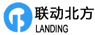操作    引用/回复总帖数 1 每页帖数 10 1/1页 1请输入验证码：您需要登录后才可以回帖 登录 | 注册技术讨论